MathScore EduFighter is one of the best math games on the Internet today. You can start playing for free!

## Nonlinear Functions - Sample Math Practice Problems

The math problems below can be generated by MathScore.com, a math practice program for schools and individual families. References to complexity and mode refer to the overall difficulty of the problems as they appear in the main program. In the main program, all problems are automatically graded and the difficulty adapts dynamically based on performance. Answers to these sample questions appear at the bottom of the page. This page does not grade your responses.

See some of our other supported math practice problems.

Give the equation of this quadratic function. It should be in the form of 'y = nx2' where n is an integer. Type x2 as x^2, etc.

 1.2.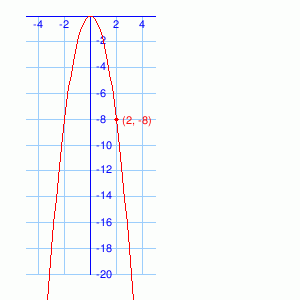### Complexity=3, Mode=cubic

Give the equation of this cubic function. It should be in the form of 'y = nx3' where n is an integer. Type x2 as x^2, etc.

 1.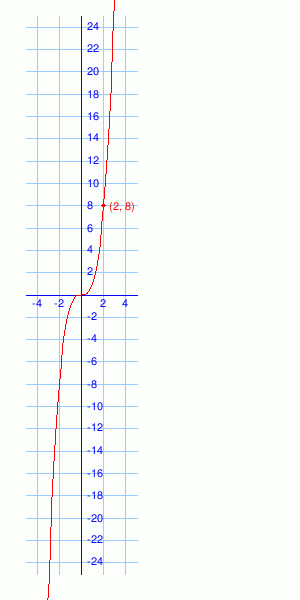2.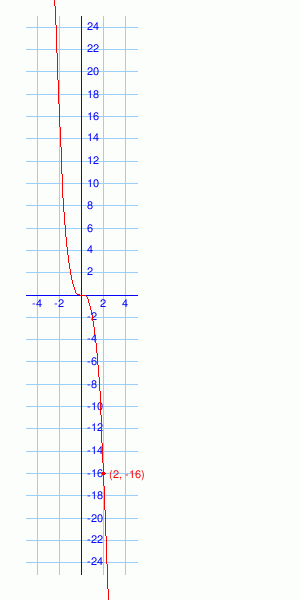### Complexity=5, Mode=mixed

Give the equation of the following functions. They may be quadratic or cubic functions. They should be of the form 'y = nx2 + b' or 'y = nx3 + b' where n and b are integers. Type x2 as x^2, etc.

 1.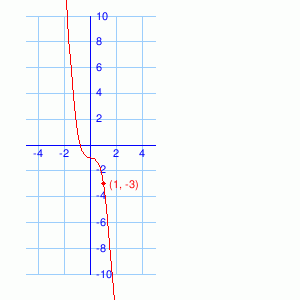2.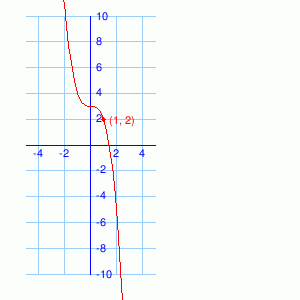Give the equation of this quadratic function. It should be in the form of 'y = nx2' where n is an integer. Type x2 as x^2, etc.

1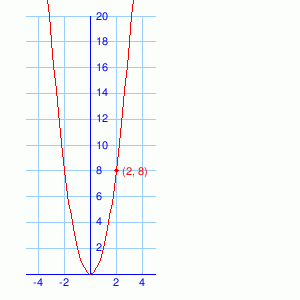Solution
The parabola opens upward so we know that, in y = nx2, n > 0.
We also know (2, 8) is on the parabola. So
8 = n * 22
8 = 4n
n = 2
So y = 2x2
2Solution
The parabola opens downward so we know that, in y = nx2, n < 0.
We also know (2, -8) is on the parabola. So
-8 = n * 22
-8 = 4n
n = -2
So y = -2x2

### Complexity=3, Mode=cubic

Give the equation of this cubic function. It should be in the form of 'y = nx3' where n is an integer. Type x2 as x^2, etc.

1Solution
The cubic function increases as x increases so we know n > 0.
(2, 8) is in the cubic function.
y = nx3
8 = n * 23
8 = 8n
n = 1
y = x3
2Solution
The cubic function decreases as x increases so we know n < 0.
(2, -16) is in the cubic function.
y = nx3
-16 = n * 23
-16 = 8n
n = -2
y = -2x3

### Complexity=5, Mode=mixed

Give the equation of the following functions. They may be quadratic or cubic functions. They should be of the form 'y = nx2 + b' or 'y = nx3 + b' where n and b are integers. Type x2 as x^2, etc.

1Solution
The function decreases as x increases and doesn't open in a direction like a parabola, so it is a cubic function of the form y = nx3 + b with n < 0.
The equation intersects the y-axis at(0, -1), so
y = nx3 + b
-1 = n * 03 + b
-1 = b
y = nx3 -1
We also know (1, -3) is in the parabola.
-3 = n * 13 -1
-3 = n -1
n = -2
y = -2x3 -1
2Solution
The function decreases as x increases and doesn't open in a direction like a parabola, so it is a cubic function of the form y = nx3 + b with n < 0.
The equation intersects the y-axis at(0, 3), so
y = nx3 + b
3 = n * 03 + b
3 = b
y = nx3 + 3
We also know (1, 2) is in the parabola.
2 = n * 13 + 3
2 = n + 3
n = -1
y = -x3 + 3